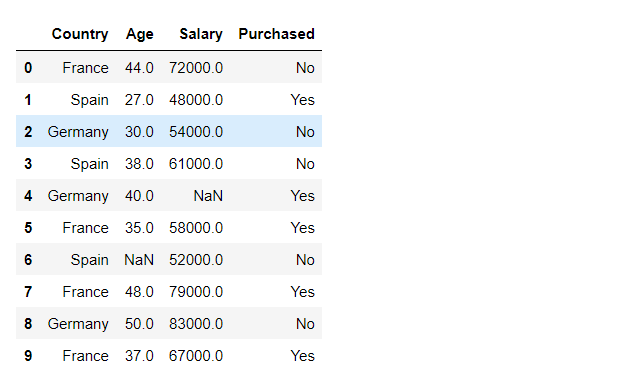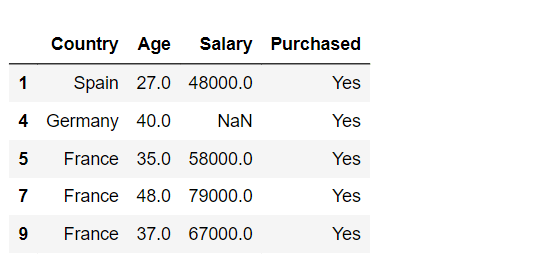GeeksforGeeks App
Open AppBrowser
Continue

# How to search a value within a Pandas DataFrame row?

In this article, we will see how to search a value within Pandas DataFrame row in Python.

### Importing Libraries and  Data

Here we are going to import the required module and then read the data file as dataframe.

The link to dataset used is here

## Python3

 `# importing pandas as ps``import` `pandas as pd` `# importing data using .read_csv() method``df ``=` `pd.read_csv(``"data.csv"``)`

Output:### Searching a Value

Here we will search the column name with in the dataframe.

Syntax : df[df[‘column_name’] == value_you_are_looking_for]

where df is our dataFrame

We will search all rows which have a value “Yes” in purchased column.

## Python3

 `df[df[``"Purchased"``] ``=``=` `"Yes"``]``# This line of code will print all rows``# which satisfy the condition df["Purchased"] == "Yes"` `# In other words df["Purchased"] == "Yes"``# will return a boolean either true or false.` `# if it returns true then we will print that``# row otherwise we will not print the row.`

Output:We can also use more than one condition to search a value. Lets see a example to find all rows which have Age value between 35 and 40 inclusive.

Syntax : df[condition]

where df is our dataFrame

## Python3

 `df[(df[``"Age"``] >``=` `35``) & (df[``"Age"``] <``=` `40``)]` `# This line of code will return all``# rows which satisfies both the conditions``# ie value of age >= 35 and value of age <= 40`

Output:My Personal Notes arrow_drop_up